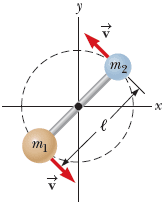1
0
watching
72
views9 Nov 2019

# A light rod of length = 1.00 m rotates about an axisperpendicular to its length and passing through its center as inthe figure below. Two particles of masses m1 = 4.95 kg and m2 =3.00 kg are connected to the ends of the rod.(a) Neglecting the mass of the rod, what is the system's kineticenergy when its angular speed is 2.10 rad/s?______J(b) Repeat the problem, assuming the mass of the rod is taken to be2.10 kg.______J

For unlimited access to Homework Help, a Homework+ subscription is required.Hubert KochLv2
12 Aug 2019

## Unlock all answers

Get 1 free homework help answer.